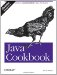# Recipe 4.5 Printing All Occurrences of a Pattern

#### Problem

You need to find all the strings that match a given regex in one or more files or other sources.

#### Solution

This example reads through a file one line at a time. Whenever a match is found, I extract it from the line and print it.

This code takes the group( ) methods from Recipe 4.3, the substring method from the CharacterIterator interface, and the match( ) method from the regex and simply puts them all together. I coded it to extract all the "names" from a given file; in running the program through itself, it prints the words "import", "java", "until", "regex", and so on:

`> jikes +E -d . ReaderIter.java > java ReaderIter ReaderIter.java import java util regex import java io Print all the strings that match given pattern from file public`

I interrupted it here to save paper. This can be written two ways, a traditional "line at a time" pattern shown in Example 4-3 and a more compact form using "new I/O" shown in Example 4-4 (the "new I/O" package is described in Chapter 10).

`import java.util.regex.*; import java.io.*; /**  * Print all the strings that match a given pattern from a file.  */ public class ReaderIter {     public static void main(String[] args) throws IOException {         // The regex pattern         Pattern patt = Pattern.compile("[A-Za-z][a-z]+");         // A FileReader (see the I/O chapter)         BufferedReader r = new BufferedReader(new FileReader(args));         // For each line of input, try matching in it.         String line;         while ((line = r.readLine( )) != null) {             // For each match in the line, extract and print it.             Matcher m = patt.matcher(line);             while (m.find( )) {                 // Simplest method:                 // System.out.println(m.group(0));                 // Get the starting position of the text                 int start = m.start(0);                 // Get ending position                 int end = m.end(0);                 // Print whatever matched.                 System.out.println("start=" + start + "; end=" + end);                 // Use CharSequence.substring(offset, end);                 System.out.println(line.substring(start, end));             }         }     } }`

##### Example 4-4. GrepNIO.java
`import java.io.*; import java.nio.*; import java.nio.channels.*; import java.nio.charset.*; import java.util.regex.*; /* Grep-like program using NIO, but NOT LINE BASED.   * Pattern and file name(s) must be on command line.  */ public class GrepNIO {     public static void main(String[] args) throws IOException {         if (args.length < 2) {             System.err.println("Usage: GrepNIO patt file [...]");             System.exit(1);         }         Pattern p = Pattern.compile(args);         for (int i=1; i<args.length; i++)             process(p, args[i]);     }     static void process(Pattern pattern, String fileName) throws IOException {         // Get a FileChannel from the given file.         FileChannel fc = new FileInputStream(fileName).getChannel( );         // Map the file's content         ByteBuffer buf = fc.map(FileChannel.MapMode.READ_ONLY, 0, fc.size( ));         // Decode ByteBuffer into CharBuffer         CharBuffer cbuf =             Charset.forName("ISO-8859-1").newDecoder( ).decode(buf);         Matcher m = pattern.matcher(cbuf);         while (m.find( )) {             System.out.println(m.group(0));         }     } }`

The NIO version shown in Example 4-4 relies on the fact that an NIO Buffer can be used as a CharSequence. This program is more general in that the pattern argument is taken from the command-line argument. It prints the same output as the previous example if invoked with the pattern argument from the previous program on the command line:

`java GrepNIO " [A-Za-z][a-z]+"  ReaderIter.java`

You might think of using \w+ as the pattern; the only difference is that my pattern looks for well-formed capitalized words while \w+ would include Java-centric oddities like theVariableName, which have capitals in nonstandard positions.

Also note that the NIO version will probably be more efficient since it doesn't reset the Matcher to a new input source on each line of input as ReaderIter does.Java Cookbook, Second Edition
ISBN: 0596007019
EAN: 2147483647
Year: 2003
Pages: 409
Authors: Ian F Darwin

Similar book on Amazon